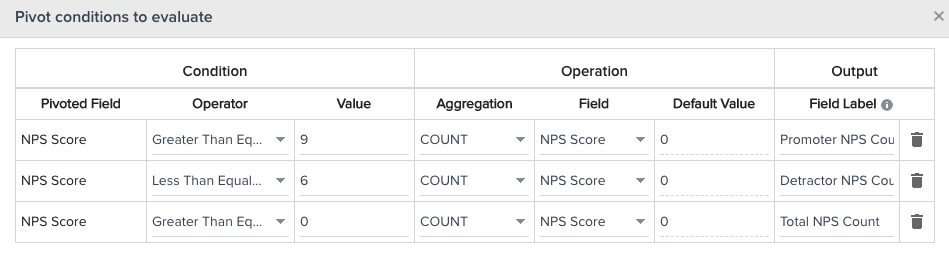# Create a rule to determine the true NPS score over a period of time

This tutorial explains the procedure to create a rule to determine the true NPS score over a period of time using Bionic Rules in Rules Engine. To achieve this, we will create a rule containing the Account and NPS score fields, and add multiple tasks to the rule. We will add a Pivot task to define the criteria for Promoters and Detractors using the NPS scores. Lastly, we will add a Transformation task to create a formula field which calculates the true NPS score.

Perform the following steps to create the required rule:

1. Navigate to Administration > Operations > Rules Engine.
2. Click +RULE.
3. In the New Rule page, select the Rule Type as Bionic.
4. Select Rule For as Account.
5. Enter the rule name and description.
6. Select a folder for the rule.1. Click NEXT.
2. Select DATASET.
3. Enter the required task name.
4. Select Native Data as the source and NPS SurveyResponse as the source object.
5. Select the Account and NPS Score fields in the Show section.
6. Apply the following filter:

NPSSurvey Response: Last Modified Date >= Subtract N Days from Rule Date  = 180

Note: In this example, we will determine the true NPS score over 180 days.1. Click SAVE and then click CANCEL.
2. Click +TASK and select Pivot.
3. Select Fetch NPS Scores as the source object.
4. Enter the required task name such as Pivot NPS.
5. In the Show section, select the Account field.
6. In the Pivot On section, select the NPS Score field.
7. In the Group By section, select the Account field.
8. To set the criteria for percentage of Promoters and Detractors using NPS Score, apply the pivot conditions as specified in the screenshot below (or as per your requirements):1. Click SAVE and then click CANCEL.
2. Click +TASK and select Transformation.
3. To calculate the true NPS, add a formula field for True NPS Calculation as specified in the screenshot below (or as per your requirements):1. Add this formula field (True NPS Calculation) and the Account field in the Show section of the transformation task.1. Click SAVE.1. Run the required rule from the listings page by clicking the RUN NOW button.

Note: To view the results, you can create a custom object and add the True NPS Calculation, NPS Name, and Account fields to it. Then you can add the Load to MDA action to this custom object (with update operation), and run the rule.

The output displays the true NPS Score over the last 180 days.